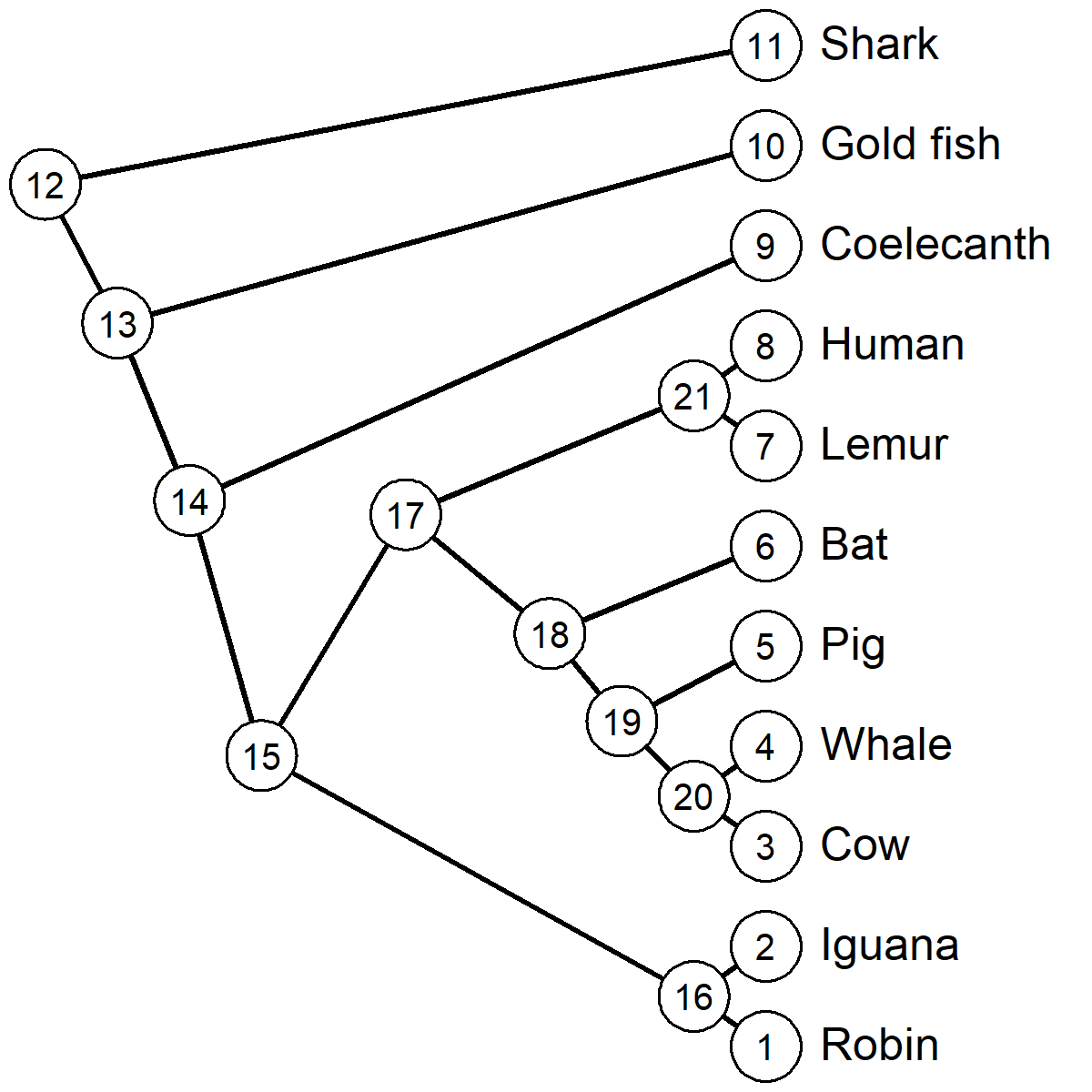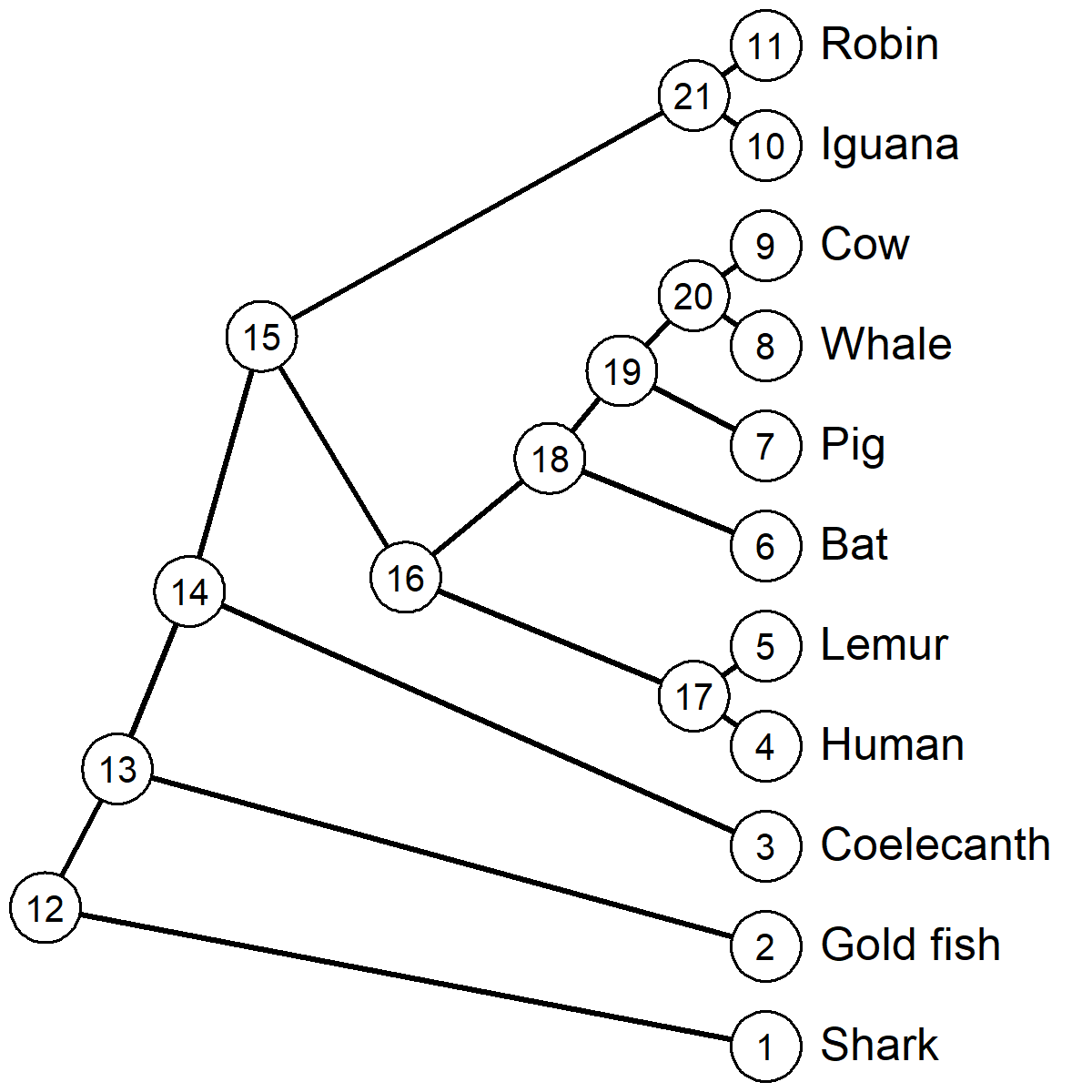### 1. Node rotation in Figure 1.1/1.2 and indices in Figure 1.3

An astute reader noticed that the node rotations & numbering of Figures 1.1/1.2 and 1.3 of Chapter 1 in an interactive R session do not match what is shown in the book chapter.

This is because all of the nodes in the plotted trees have been rotated relative to the tree that should have been created by ape::read.tree based on the Newick text string represented in the book.

In other words, either Figure 1.3 should’ve been as follows.

library(ape)
library(phytools)
text.string<-
"(((((Robin,Iguana),((((Cow,Whale),Pig),Bat),(Lemur,Human))),Coelecanth),Gold_fish),Shark);"
labelnodes(1:(Ntip(vert.tree)+vert.tree$Nnode), 1:(Ntip(vert.tree)+vert.tree$Nnode),
interactive=FALSE,cex=0.8)Figure 1.3 as it should have been shown in the book.

Or, alternatively, the following R code could have been provided

text.string<-
"(Shark,(Gold_fish,(Coelecanth,(((Human,Lemur),(Bat,(Pig,(Whale,Cow)))),(Iguana,Robin)))));"
labelnodes(1:(Ntip(vert.tree)+vert.tree$Nnode), 1:(Ntip(vert.tree)+vert.tree$Nnode),
interactive=FALSE,cex=0.8)Figure 1.3 as represented in the book, from the indicated corrected R code.

To get from one Newick string to the other, it’s possible to run the following code.

vert.tree<-read.tree(
text="(((((Robin,Iguana),((((Cow,Whale),Pig),Bat),(Lemur,Human))),Coelecanth),Gold_fish),Shark);")
vert.tree<-phytools::rotateNodes(vert.tree,"all")
write.tree(vert.tree)
##  "(Shark,(Gold_fish,(Coelecanth,(((Human,Lemur),(Bat,(Pig,(Whale,Cow)))),(Iguana,Robin)))));"

Ta-da.